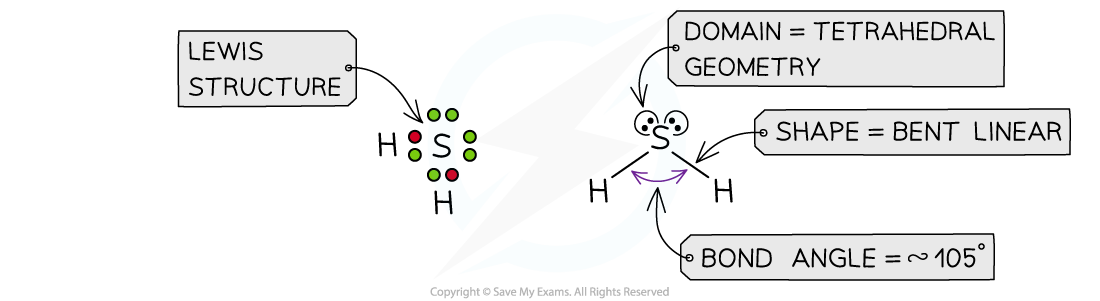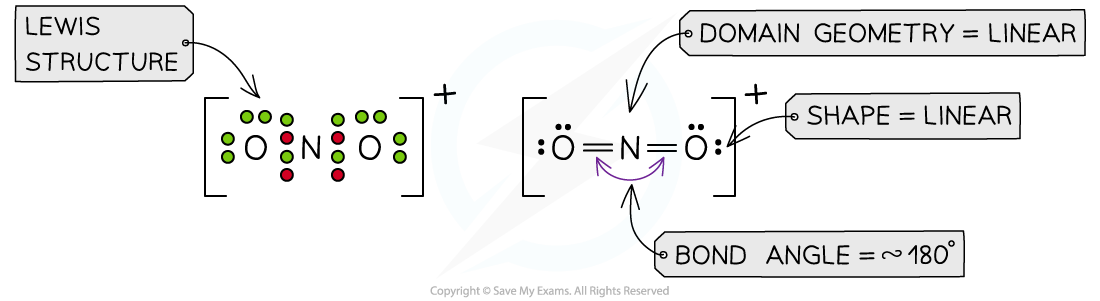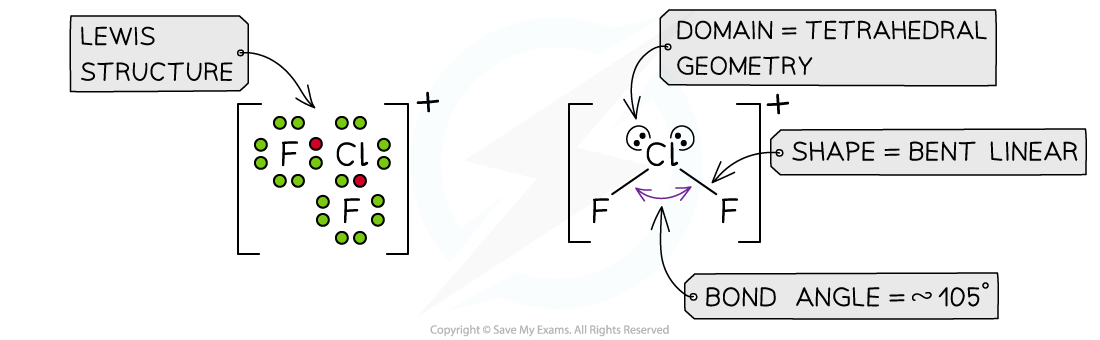# IB DP Chemistry: SL复习笔记4.2.3 Predicting Molecular Shapes

### Predicting Shapes & Bond Angles

• Before you predict the shape of any molecule work out the Lewis structure to determine the number of bonding and lone pairs
• Apply the VSEPR rules and you should be successful in deducing the correct shape and bond angle

#### Worked Example

Predict the domain geometry, shape and bond angle in the following molecules or ions:

1. H2S
2. NH2Cl
3. NO2+
4. ClF2+

Answer 1: The total number of valence electrons in H2S is = 1 + 1 + 6 = 8, so there are four pairs of electrons around S

Hydrogen only forms one bond, so there are two bonding pairs and two lone pairs:Answer 2: The total number of valence electrons in NH2Cl is = 5 + 1 + 1 + 7 = 14Answer 3: The total number of valence electrons in NO2+= 5 + 6 + 6 -1 = 16  (subtracting one for the positive charge)Answer 4: The total number of valence electrons in ClF2+= 7 + 7 + 7 -1 = 20  (subtracting one for the positive charge)#### Exam Tip

For Standard Level Chemistry you are only required to know the shape of molecules up to four electron domains.